# Class 8 Maths NCERT Solutions for Chapter – 14 Factorisation Ex – 14.1

## Factorisation

Question 1.
Find the common factors of the given terms :
(i)
12x, 36
(ii) 2y, 22xy
(iii) 14pqr, 28p2q2
(iv) 2x, 3x2, 4
(v) 6abc, 24ab2,12a2b
(vi) 16x3, – 4x2, 32x
(vii) 10pq, 20qr, 30rp
(viii) 3x2y3, 10x3y2, 6x2y2z

Solution:
(i) The numerical coefficients in the given monomials are 12 and 36.
The highest common factor of 12 and 36 is 12.
But there is no common literal appearing in the given monomials 12x and 36.
∴ The highest common factor =12

(ii) The numerical coefficients of the given monomials are 2 and 22.
The highest common factor of 2 and 22 is 2.
The common literal appearing in the given monomials is y.
The smallest power of y in the two monomials =1
The monomial of common literals with smallest powers = y
∴ The highest common factor = 2y

(iii) The numerical coefficients of the given monomials are 14 and 28.
The highest common factor of 14 and 28 is 14.
The common literals appearing in the given monomials are p and q.
The smallest power of p and q in the two monomials = 1
The monomial of common literals with smallest powers pq
∴ The highest common factor = 14pq

(iv) The numerical coefficients of the given monomials are 2, 3, and 4.
The highest common factor of 2, 3 and 4 is 1.
There is no common literal appearing in the three monomials.
The highest common factor = 1

(v) The numerical coefficients of the given monomials are 6, 24 and 12.
The highest common factor of 6, 24 and 12 is 6.
The common literals appearing in the three monomials are a and b.
The smallest power of a in the three monomials = 1
The smallest power of b in the three monomials 1
The monomials of common literals with smallest powers = ab
Hence, the highest common factor =6 ab

(vi) The numerical coefficients of the given monomials are 16, 4 and 32.
The highest common factor of 16, 4 and 32 is 4.
The common literal appearing in the three monomials is x.
The smallest power of x in the three monomials =1
The monomial of common literal with smallest power = x
Hence, the highest common factor = 4x

(vii) The numerical coefficients of the given monomials are 10,20 and 30.
The highest common factor of 10, 20 and 30 is 10
There is no common literal appearing in the three monomials.
Hence, the highest common factors =10.

(viii) The numerical coefficients of the given monomials are 3, 10 and 6.
The highest common factor of 3, 10 and 6 is 1.
The common literals appearing in the three monomials are x and y.
The smallest power of x in the three monomials = 2
The smallest power of y in the three monomials = 2
The monomial of common literals with smallest powers = x2y2
Hence, the highest common factor = x2y2

Question 2.
Factorise the following expressions
(i) 7x – 42
(ii) 6p – 12q
(iii) 7a2 + 14a
(iv) -16z + 20z2
(v) 20l2 m + 30alm
(vi) 5x2y – 15xy2
(vii) 10a2 + 15b2 + 20c2
(viii) -4a2 + 4ab – 4ca
(ix) x2yz + xy2z + xyz2
(x) ax2y + bxy2 + cxyz

Solution: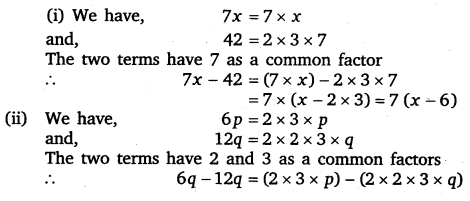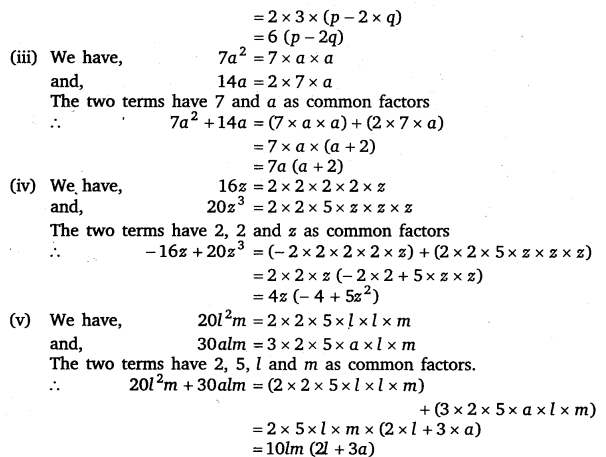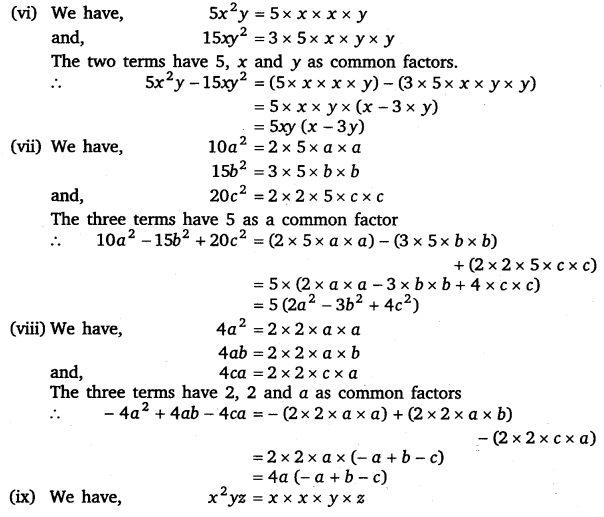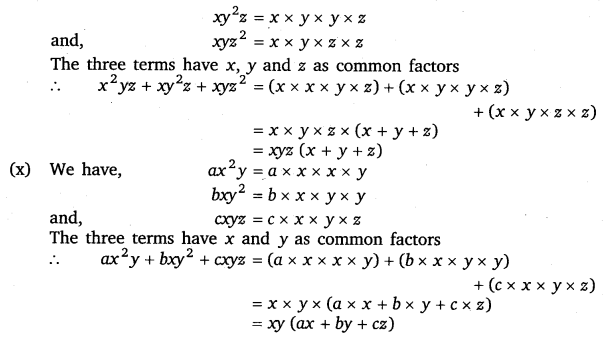Question 3.
Factorise :
(i)
x2 + xy + 8x + 8y
(ii) 15xy – 6x + 5y – 2
(iii) ax + bx – ay – by
(iv) 15pq + 15 + 9q + 25p
(v) z – 7 + 7xy – xyz

Solution: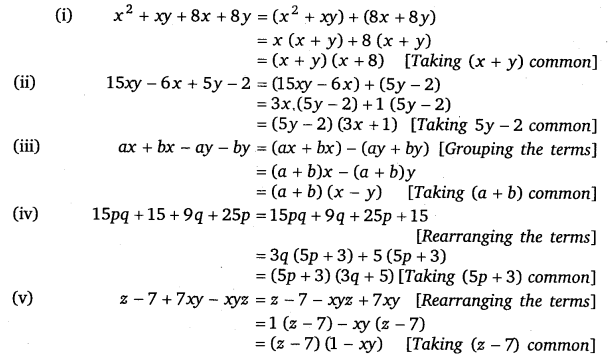#### Class 8 Maths NCERT Solutions for Chapter – 14 Factorisation Ex – 14.2

Lorem ipsum dolor sit amet, consectetur adipiscing elit. Phasellus cursus rutrum est nec suscipit. Ut et ultrices nisi. Vivamus id nisl ligula. Nulla sed iaculis ipsum.

Company Name